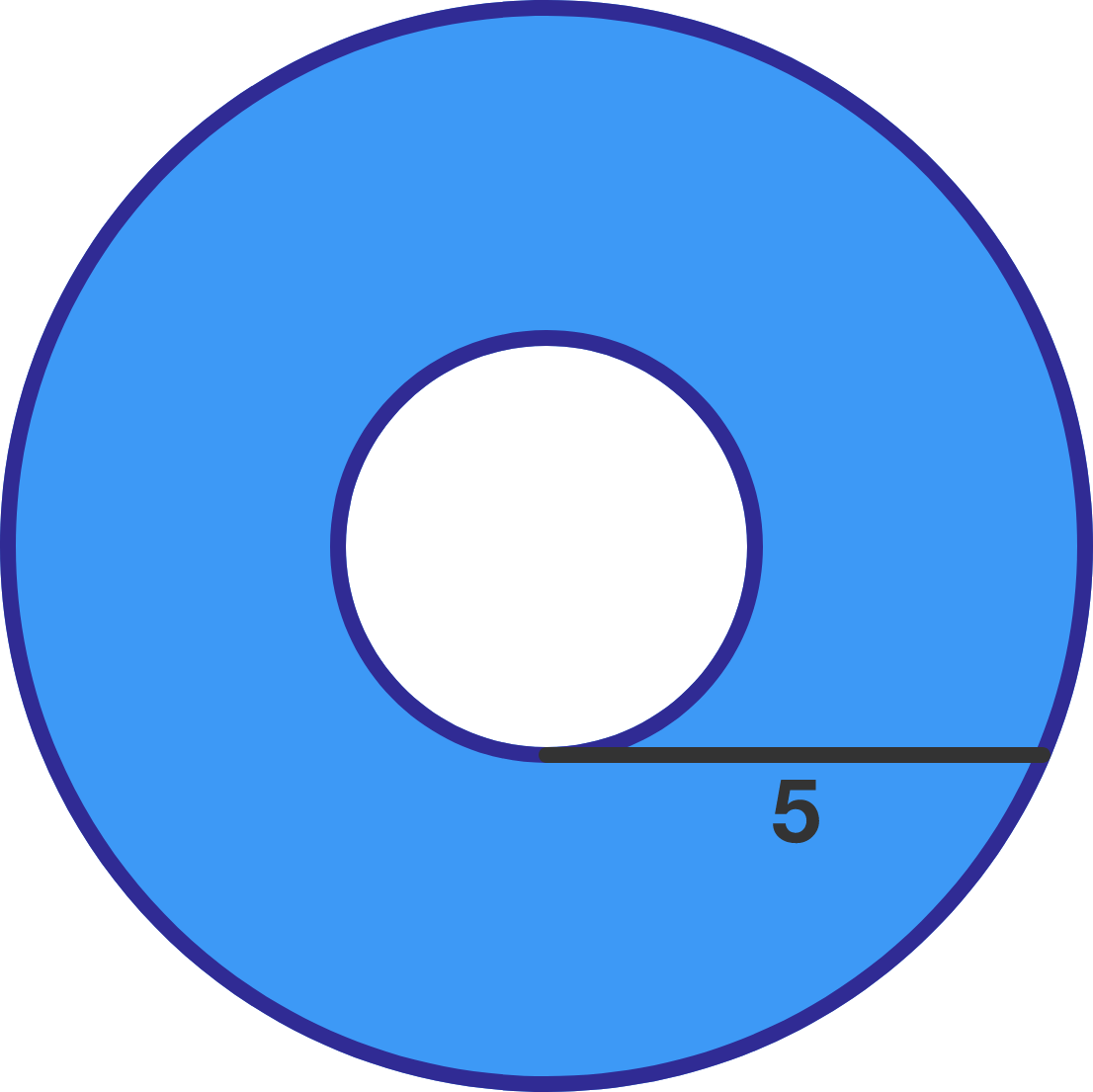# Problems of the Week

Contribute a problem

# 2018-02-05 Basic

The density of helium is lower than that of air. As a result, when a helium balloon is released, it floats up into the sky (see buoyancy).

What happens to the volume of the helium balloon as it rises up?

Assume

• the balloon is made of an excellent thermal insulator, so no heat enters or leaves the balloon;
• the balloon is tied properly so that gases cannot enter or leave the balloon.$\large \begin{array}{c}& 0 & & 0 & & 0 & = 6 \end{array}$

Using basic arithmetic operations $(+,\ -,\ \times,\ \div),$ parentheses, and any other operations on the left side of the equal sign, can you make this equality hold true?

Note: Some of the other operations you might try are the trigonometric functions $\big(\sin(\cdot)$, $\cos(\cdot)$, and $\tan(\cdot)\big)$, factorial $!$, floor $\lfloor \cdot \rfloor$, and ceiling $\lceil \cdot \rceil$. You can put in as many operations as you like, but you can't put in any additional numbers or digits!

Monty prefers to eat his ice cream in a silver bowl compared to an otherwise identical glass bowl. In which bowl will the ice cream melt faster?$\large 1\times2\times3\times\cdots\times2018$ My computer solves for the above product and finds its digit sum (sum of all its digits). It then repeats this process of calculating the digit sum until only one digit remains.

What digit is that?The black segment in the diagram is tangent to the inner circle, ends on the outer circle, and is length 5.

Find the area of the circular ring (the blue region).

×

Problem Loading...

Note Loading...

Set Loading...